# Truncated stick breaking in greta¶

Here, we briefly discuss truncated stick breaking (TSB). We use TSB as a finite-dimensional alternative to an infinite dimensional prior over the number of mixture components in a mixture model. We do the analysis in greta. TSB allows us to use continuous parameters entirely, which in turn allows us to use Hamiltonian Monte Carlo (and since most probabilistic programming languages do not allow discrete paramters). Also, in practice, working with continuous parameters is way easier than discrete ones (as with the Chinese restaurant process).

We first try to use TSB with a mixture of univariate normals, and then with Poisson variables.

Some required libraries:

In :
suppressMessages(library("greta"))
suppressMessages(library("tensorflow"))

In :
suppressMessages(library("tidyverse"))
suppressMessages(library("MASS"))
suppressMessages(library("bayesplot"))
suppressMessages(library("caret"))
options(repr.plot.width=8, repr.plot.height=3)


Reproducibility!

In :
options(repr.plot.width=8, repr.plot.height=3)
set.seed(23)


### Normal data¶

We first create some data. We create a data set of $1000$ observations per mixture component.

In :
N <- 1000
K <- 3


We set the means of the mixture components to $\boldsymbol \mu = \{ -2, 0, 2\}$. We use a single standard deviations $\sigma=0.25$.

In :
mus <- c(-2, 0, 2)
sd <- .25


Then we create latent indicator variables $Z$ and sample normal data w.r.t to the cluster indicator.

In :
data <- vector(length = N * K)
Z <- factor(rep(seq(K), each=N))
for (k in seq(K)) {
idx <- seq(N) + ((k - 1) * N)
data[idx] <- rnorm(N, mus[k], sd)
}

In :
data.frame(data=data, idx=as.factor(rep(seq(K), each=N))) %>%
ggplot(aes(data, fill=idx)) +
geom_histogram(bins=30, position = "dodge") +
scale_fill_viridis_d("Component", alpha = 1, begin=.3, end=.8) +
theme_minimal()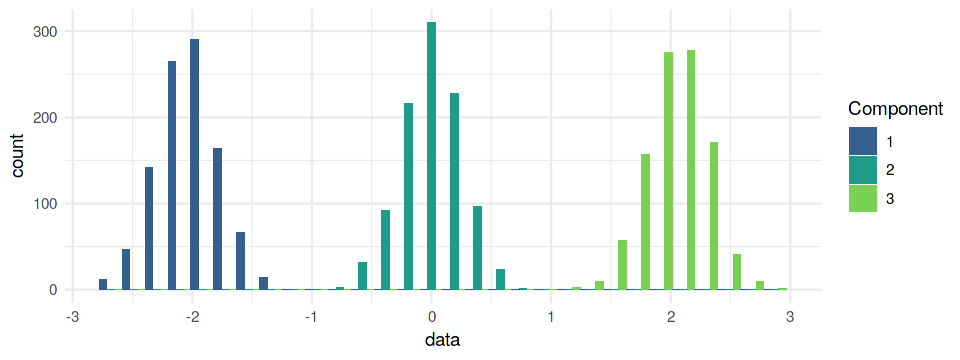For our data set it should be fairly easy to find the correct number of clusters ($3$). For the TSB, we need to set the number of clusters to a sufficiently high $K$, to achieve a negligibly small error in comparison to a "true" infinite dimensional prior.

See for instance https://projecteuclid.org/euclid.bj/1551862850 for a theoretical and practical justification for the truncation.

In :
K <- 10


We will only try to estimate the vector of mean values of the Gaussians. In order to avoid non-identifiability, we can use a small trick: we create a prior of means of length $K$ and, use the cumulative sum (cumsum), and ensure that the mean values are sorted and increasing in value. For instance:

In :
x <- c(runif(1, -1, 1), runif(5, 0, 1))
cumsum(x)

1. 0.593454854562879
2. 0.856230824021623
3. 1.22660479671322
4. 2.02785729477182
5. 2.67813442042097
6. 3.13572235242464

In greta that is:

In :
prior_mu_ordered <- cumsum(
c(greta::variable(lower = -5, upper = 5),
greta::variable(lower = 0, upper = 5, dim = K - 1)))


Then we set a prior over the mixing weights. For this, as mentioned before, we use stick breaking. Luckily LaplacesDemon has a function for the sticks.

In :
stick_breaking <- function(theta) {
LaplacesDemon::Stick(theta)
}

# note the K - 1 which is required for LaplacesDemon::Stick (yes it's dumb)
prior_stick <- greta::beta(1, 1, dim = K - 1)
prior_weights <- stick_breaking(prior_stick)


Then we set the mixture distribution and sample from the posterior. This is a little annoying in R, cause we need to do it manually.

In :
greta::distribution(data) <- greta::mixture(
greta::normal(prior_mu_ordered, .25),
greta::normal(prior_mu_ordered, .25),
greta::normal(prior_mu_ordered, .25),
greta::normal(prior_mu_ordered, .25),
greta::normal(prior_mu_ordered, .25),
greta::normal(prior_mu_ordered, .25),
greta::normal(prior_mu_ordered, .25),
greta::normal(prior_mu_ordered, .25),
greta::normal(prior_mu_ordered, .25),
greta::normal(prior_mu_ordered, .25),
weights = prior_weights
)

In :
mod <- greta::model(prior_stick, prior_weights, prior_mu_ordered)

In :
samples <- greta::mcmc(mod, n_cores = 1, chains = 1)

    warmup ====================================== 1000/1000 | eta:  0s
sampling ====================================== 1000/1000 | eta:  0s


Let's see if we could identify the components.

In :
bayesplot::mcmc_hist(samples, regex_pars = "weights")

stat_bin() using bins = 30. Pick better value with binwidth.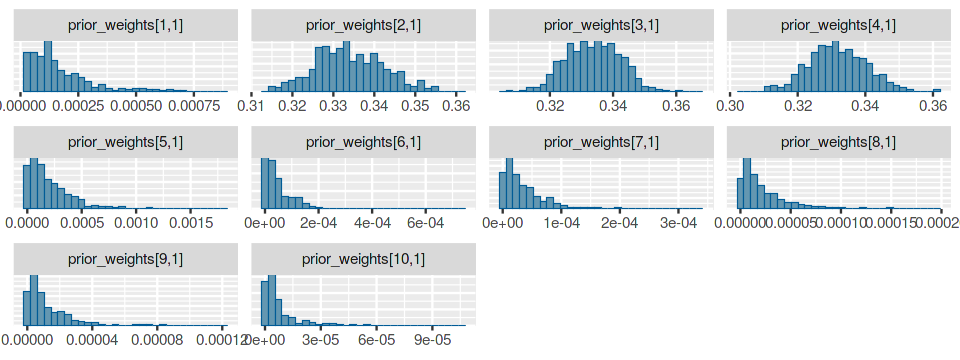Looks like it suffices to use three components (as only three components of the weight posterior significant weight). Now, let's see if we could identify the means correctly. Since we only use components , we only consider these means.

In :
bayesplot::mcmc_intervals(samples, regex_pars = "mu")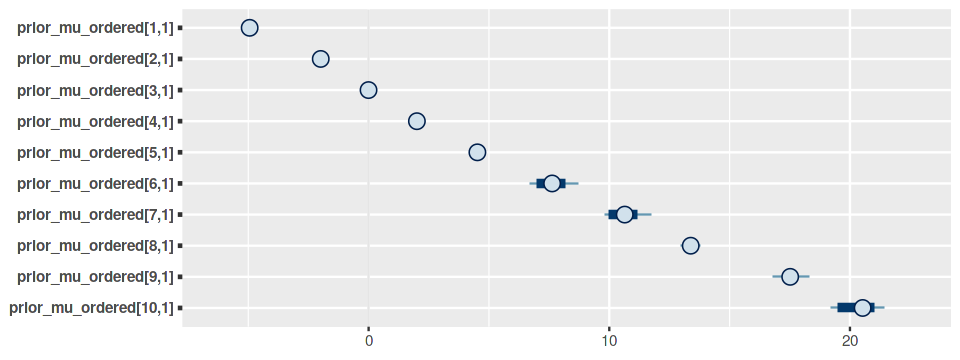That worked great. We were perfectly able to recover the correct number of components. Now, let's cluster them. We only cluster with the componentes 2, 3 and 4, since our posterior weights suggest, these are the important ones.

In :
posterior_matrix <- as.matrix(samples)
posterior_mus <- posterior_matrix[,sprintf("prior_mu_ordered[%i,1]", c(2, 3, 4))]


Here we assign each point its most likely assignment

In :
clusters <- vector(length = length(data))
for (i in seq(data)) {
probs <- apply(posterior_mus, 2, function(.) mean(dnorm(data[i], ., .25)))
clusters[i] <- which.max(probs)
}


Finally we compute a confusion matrix to check our predictions.

In :
caret::confusionMatrix(factor(clusters), Z)

Confusion Matrix and Statistics

Reference
Prediction    1    2    3
1 1000    0    0
2    0 1000    0
3    0    0 1000

Overall Statistics

Accuracy : 1
95% CI : (0.9988, 1)
No Information Rate : 0.3333
P-Value [Acc > NIR] : < 2.2e-16

Kappa : 1

Mcnemar's Test P-Value : NA

Statistics by Class:

Class: 1 Class: 2 Class: 3
Sensitivity            1.0000   1.0000   1.0000
Specificity            1.0000   1.0000   1.0000
Pos Pred Value         1.0000   1.0000   1.0000
Neg Pred Value         1.0000   1.0000   1.0000
Prevalence             0.3333   0.3333   0.3333
Detection Rate         0.3333   0.3333   0.3333
Detection Prevalence   0.3333   0.3333   0.3333
Balanced Accuracy      1.0000   1.0000   1.0000

### Poisson data¶

Next we try a Poisson mixture.

In :
N <- 1000
K <- 3
mus <- exp(c(0, 1, 2))

In :
data <- vector(length = N * K)
Z <- factor(rep(seq(K), each=N))
for (k in seq(K)) {
idx <- seq(N) + ((k - 1) * N)
data[idx] <- rpois(N, mus[k])
}

In :
data.frame(data=data, idx=as.factor(rep(seq(K), each=N))) %>%
ggplot(aes(data, fill=idx)) +
geom_histogram(bins=30, position = "dodge") +
scale_fill_viridis_d("Component", alpha = 1, begin=.3, end=.8) +
theme_minimal()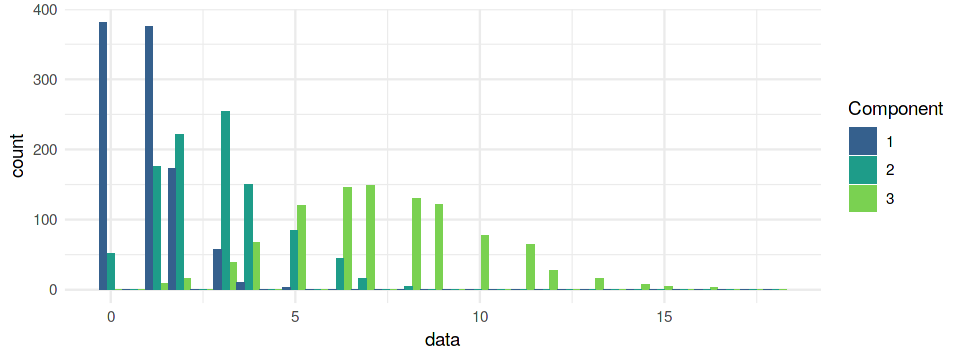We truncate the DP at $K=10$ as before and define the model.

In :
K <- 10


We can use the same the trick from before. Since we exponentiate the means later, we don't need to care about negative values.

In :
prior_mu_ordered <- cumsum(
c(greta::variable(lower = -5, upper = 3),
greta::variable(lower = 0, upper = 3, dim = K - 1)))

In :
greta::distribution(data) <- greta::mixture(
greta::poisson(exp(prior_mu_ordered)),
greta::poisson(exp(prior_mu_ordered)),
greta::poisson(exp(prior_mu_ordered)),
greta::poisson(exp(prior_mu_ordered)),
greta::poisson(exp(prior_mu_ordered)),
greta::poisson(exp(prior_mu_ordered)),
greta::poisson(exp(prior_mu_ordered)),
greta::poisson(exp(prior_mu_ordered)),
greta::poisson(exp(prior_mu_ordered)),
greta::poisson(exp(prior_mu_ordered)),
weights = prior_weights
)

In :
mod <- greta::model(prior_stick, prior_weights, prior_mu_ordered)

In :
samples <- greta::mcmc(mod, chains = 1, n_cores = 1)

    warmup ====================================== 1000/1000 | eta:  0s
sampling ====================================== 1000/1000 | eta:  0s


How many components do we need?

In :
bayesplot::mcmc_hist(samples, regex_pars = "weights")

stat_bin() using bins = 30. Pick better value with binwidth.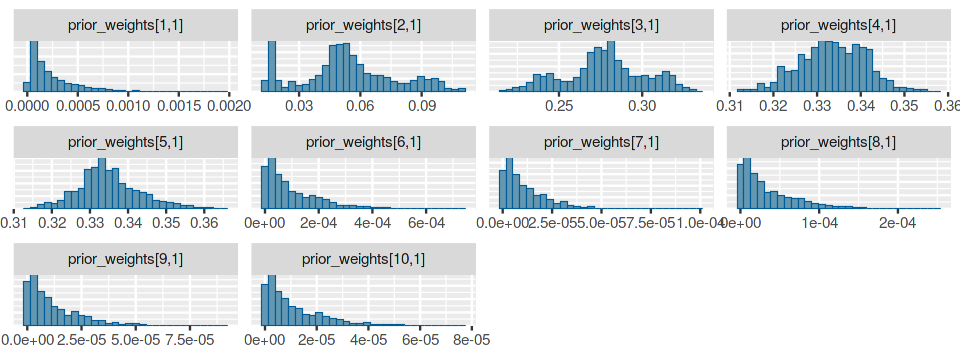Here, it's not so clear anymore. It looks as if we should use four components (so one too many), but this could be due to poor prior choice. What about the means?

In :
bayesplot::mcmc_intervals(samples, regex_pars = "mu")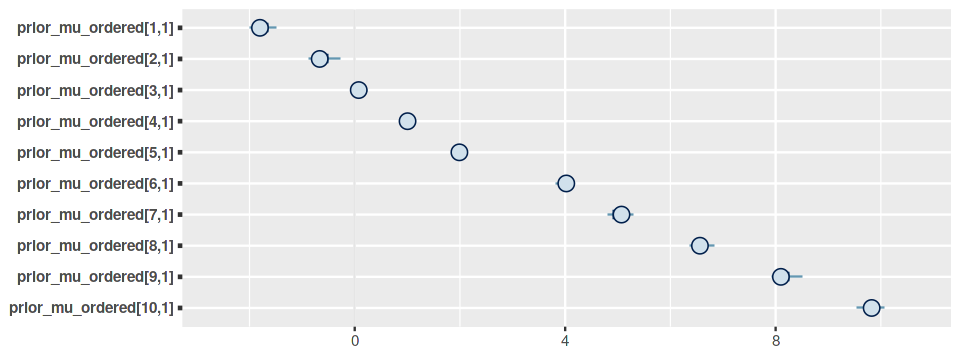Finally, let's have a look at the posterior assignments of the data points to the components again. In order to keep the cluster assignments (2, 3, 4, 5) the same as in our original assignment, we pick the second component last. By that when we call which.max it will return the correct indexes for the cluster, i.e. assignment to cluster 3 will be index 1 which corresponds to the cluster in our original assignment. Since we have four clusters to consider, the new cluster (component 2) will get index 4.

In :
posterior_matrix <- as.matrix(samples)
posterior_mus <- posterior_matrix[,sprintf("prior_mu_ordered[%i,1]", c(3, 4, 5, 2))]

In :
clusters <- vector(length = length(data))
for (i in seq(data)) {
probs <- apply(posterior_mus, 2, function(.) mean(dpois(data[i], exp(.))))
clusters[i] <- which.max(probs)
}


Since we have four clusters to consider now, we need to relevel our true latent assigments.

In :
caret::confusionMatrix(factor(clusters), factor(Z, levels=c(levels(Z), 4)))

Confusion Matrix and Statistics

Reference
Prediction   1   2   3   4
1 375 175   9   0
2 241 625 122   0
3   3 149 869   0
4 381  51   0   0

Overall Statistics

Accuracy : 0.623
95% CI : (0.6054, 0.6404)
No Information Rate : 0.3333
P-Value [Acc > NIR] : < 2.2e-16

Kappa : 0.4725

Mcnemar's Test P-Value : NA

Statistics by Class:

Class: 1 Class: 2 Class: 3 Class: 4
Sensitivity            0.3750   0.6250   0.8690       NA
Specificity            0.9080   0.8185   0.9240    0.856
Pos Pred Value         0.6708   0.6326   0.8511       NA
Neg Pred Value         0.7440   0.8136   0.9338       NA
Prevalence             0.3333   0.3333   0.3333    0.000
Detection Rate         0.1250   0.2083   0.2897    0.000
Detection Prevalence   0.1863   0.3293   0.3403    0.144
Balanced Accuracy      0.6415   0.7218   0.8965       NA

Obviously, the assignment suffers from the fact that the Poisson components are not very well separated. However, the clustering is still fairly good.